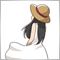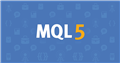# Conversion of ATR Pips in MT4 to MT558

```//+------------------------------------------------------------------+
//|           ATR Percentage S/L-T/P .mq4
//|                                                                  |
//|                                                                  |
//+------------------------------------------------------------------+

#property indicator_chart_window
#property indicator_buffers 4
#property indicator_plots 4

#property  indicator_color1  clrRed
#property indicator_type1   DRAW_LINE
#property indicator_style1  STYLE_SOLID
#property indicator_width1  1

#property  indicator_color2  clrGreen
#property indicator_type2   DRAW_LINE
#property indicator_style2  STYLE_SOLID
#property indicator_width2  1

#property  indicator_color3  clrRed
#property indicator_type3   DRAW_LINE
#property indicator_style3  STYLE_SOLID
#property indicator_width3  1

#property  indicator_color4  clrGreen
#property indicator_type4   DRAW_LINE
#property indicator_style4  STYLE_SOLID
#property indicator_width4  1

//+-----------------------------------+
//|  INDICATOR INPUT PARAMETERS       |
//+-----------------------------------+
extern int periods = 14;
extern double percentSL = 1.5;
extern double percentTP1 = 2;
extern double percentTP2 = 3;
extern double percentTP3 = 4;
extern int corner = 4;

int pipMult = 10000;

//---- Indicator buffers
double buffera[];
double bufferb[];
double bufferc[];
double bufferd[];

string prefixa = "";
string prefixb = "";
string prefixc = "";
string prefixd = "";

//---- Declaration of integer variables of data starting point
int min_rates_total;

//+------------------------------------------------------------------+
//| Custom indicator initialization function                         |
//+------------------------------------------------------------------+
int OnInit()
{
if (StringFind(Symbol(),"JPY",0) != -1)
{
pipMult = 100;
}
{
int percentagea = percentSL*100;
prefixa = percentagea + "% of ";
}
{
int percentageb = percentTP1*100;
prefixb = percentageb + "% of ";
}
{
int percentagec = percentTP2*100;
prefixc = percentagec + "% of ";
}
{
int percentaged = percentTP3*100;
prefixd = percentaged + "% of ";
}

min_rates_total = int(periods+1);

SetIndexBuffer(0,buffera);
SetIndexBuffer(1,bufferb);
SetIndexBuffer(2,bufferc);
SetIndexBuffer(3,bufferd);

//--- setting buffer arrays as timeseries
ArraySetAsSeries(buffera,true);
ArraySetAsSeries(bufferb,true);
ArraySetAsSeries(bufferc,true);
ArraySetAsSeries(bufferd,true);

PlotIndexSetString(0,PLOT_LABEL,"ATR (" + periods + ")");

ObjectCreate(0,"label_ATRinPipsSL", OBJ_LABEL, 0, 0, 0);
ObjectCreate(0,"label_ATRinPipsTP1", OBJ_LABEL, 0, 0, 0);
ObjectCreate(0,"label_ATRinPipsTP2", OBJ_LABEL, 0, 0, 0);
ObjectCreate(0,"label_ATRinPipsTP3", OBJ_LABEL, 0, 0, 0);

ObjectSetInteger(0, "label_ATRinPipsSL", OBJPROP_CORNER, corner);
ObjectSetInteger(0, "label_ATRinPipsSL", OBJPROP_XDISTANCE, 5);
ObjectSetInteger(0, "label_ATRinPipsSL", OBJPROP_YDISTANCE, 15);

ObjectSetInteger(0, "label_ATRinPipsTP1", OBJPROP_CORNER, corner);
ObjectSetInteger(0, "label_ATRinPipsTP1", OBJPROP_XDISTANCE, 5);
ObjectSetInteger(0, "label_ATRinPipsTP1", OBJPROP_YDISTANCE, 18+10);

ObjectSetInteger(0, "label_ATRinPipsTP2", OBJPROP_CORNER, corner);
ObjectSetInteger(0, "label_ATRinPipsTP2", OBJPROP_XDISTANCE, 5);
ObjectSetInteger(0, "label_ATRinPipsTP2", OBJPROP_YDISTANCE, 18+24);

ObjectSetInteger(0, "label_ATRinPipsTP3", OBJPROP_CORNER, corner);
ObjectSetInteger(0, "label_ATRinPipsTP3", OBJPROP_XDISTANCE, 5);
ObjectSetInteger(0, "label_ATRinPipsTP3", OBJPROP_YDISTANCE, 18+38);

//---- performing the shift of beginning of indicator drawing
PlotIndexSetInteger(0,PLOT_DRAW_BEGIN,min_rates_total);
//---- setting the indicator values that won't be visible on a chart
PlotIndexSetDouble(0,PLOT_EMPTY_VALUE,EMPTY_VALUE);
//----
return(INIT_SUCCEEDED);
}

//+------------------------------------------------------------------+
//| Custom indicator iteration function                              |
//+------------------------------------------------------------------+

int OnCalculate(
const int rates_total,    // amount of history in bars at the current tick
const int prev_calculated,// amount of history in bars at the previous tick
const datetime &time[],
const double &open[],
const double& high[],
const double& low[],
const double &close[],    // price array of price lows for the indicator calculation
const long &tick_volume[],
const long &volume[],
)
{

////////////////////////////////
//---- checking the number of bars to be enough for calculation
if(rates_total<min_rates_total)return(0);

//---- Declaration of integer variables
int first,bar;

if(prev_calculated>rates_total || prev_calculated<=0) // checking for the first start of calculation of an indicator
first=min_rates_total; // starting index for calculation of all bars
else first=prev_calculated-1; // starting number for calculation of new bars

//---- main indicator calculation loop
for(bar=first; bar<rates_total; bar++)
//---- main indicator calculation loop

for(bar=first; bar<rates_total; bar++){

double stopLossa = MathCeil(pipMult * percentSL * (iATR(Symbol(),PERIOD_CURRENT,periods)));
double stopLossb = MathCeil(pipMult * percentTP1 * (iATR(Symbol(),PERIOD_CURRENT,periods)));
double stopLossc = MathCeil(pipMult * percentTP2 * (iATR(Symbol(),PERIOD_CURRENT,periods)));
double stopLossd = MathCeil(pipMult * percentTP3 * (iATR(Symbol(),PERIOD_CURRENT,periods)));

buffera[bar] = iClose(Symbol(),PERIOD_CURRENT,bar) +percentSL  * iATR(Symbol(),PERIOD_CURRENT,periods);
bufferb[bar] = iClose(Symbol(),PERIOD_CURRENT,bar) +percentTP1 * iATR(Symbol(),PERIOD_CURRENT,periods);
bufferc[bar] = iClose(Symbol(),PERIOD_CURRENT,bar) +percentTP2 * iATR(Symbol(),PERIOD_CURRENT,periods);
bufferd[bar] = iClose(Symbol(),PERIOD_CURRENT,bar)+percentTP3 * iATR(Symbol(),PERIOD_CURRENT,periods);
}

//-----Comment(prefix, "ATR (", periods, "): ", buffer, " pips");
string texta = prefixa+ "ATR ("+ periods+ ") : S/L- "+ DoubleToString(buffera,0)+" pips";
string textb = prefixb+ "ATR ("+ periods+ ") : 1st-P/T- "+ DoubleToString(bufferb,0)+" pips";
string textc = prefixc+ "ATR ("+ periods+ ") : 2nd-P/T- "+ DoubleToString(bufferc,0)+" pips";
string textd = prefixd+ "ATR ("+ periods+ ") : 3rd-P/T- "+ DoubleToString(bufferd,0)+" pips";

ObjectSetString(0,"label_ATRinPipsSL",OBJPROP_TEXT,texta);
ObjectSetString(0,"label_ATRinPipsTP1",OBJPROP_TEXT,textb);
ObjectSetString(0,"label_ATRinPipsTP2",OBJPROP_TEXT,textc);
ObjectSetString(0,"label_ATRinPipsTP3",OBJPROP_TEXT,textd);

return(0);
}
//+------------------------------------------------------------------+

//+------------------------------------------------------------------+
//| Custor indicator deinitialization function                       |
//+------------------------------------------------------------------+
//int OnDeinit()
//  {
//   ObjectDelete(0,"label_ATRinPipsSL");
//   ObjectDelete(0,"label_ATRinPipsTP1");
//   ObjectDelete(0,"label_ATRinPipsTP2");
//   ObjectDelete(0,"label_ATRinPipsTP3");
//   return(0);
//  }```
Hi, I am trying to convert the ATR Pips that I used in MQL4 to the MQL5 language. So far there were no error already on compilation but their is no plot that is being shown when being attached to the MetaTrader. Any help with the code will be greatly appreciated.715

There are a lot of things to go into, but if you modify the way you calculate ATR, you might be a little closer to the goal.

https://www.mql5.com/en/docs/indicators/iatr

The important part of the linked sample program is the following.

```handle=iATR(name,period,atr_period);

CopyBuffer(ind_handle,0,0,amount,values)```Documentation on MQL5: Technical Indicators / iATR
• www.mql5.com
//|                                                    Demo_iATR.mq5 | //|                        Copyright 2011, MetaQuotes Software Corp. | //|                                              https://www.mql5.com | "The method of creation of the handle is set through the 'type' parameter (function type...23798

`double … percentSL * (iATR(Symbol(),PERIOD_CURRENT,periods)));`

Perhaps you should read the manual, especially the examples.
How To Ask Questions The Smart Way. 2004